# An inverse three spectra problem for Sturm–Liouville operators

## Abstract

In this paper, we consider the inverse three spectra problems of recovering the Sturm–Liouville equation by the spectra of the Neumann–Dirichlet boundary value problem on $$[0,1]$$, the Neumann–Robin problem on $$[0,1/2]$$, and the Robin–Dirichlet problem on $$[1/2,1]$$, where the two Robin parameters at the interior node $$x=1/2$$ are considered to be different. The algorithm of construction is presented and sufficient conditions for three sequences to be the spectral data of the mentioned boundary problems are given.

## 1 Introduction

In this paper, we are concerned with the inverse three spectra problem for the Sturm–Liouville equation. Consider the operator $$L:=L(a,b;h_{a},h_{b};q)$$ defined as

$$Ly=-y^{\prime\prime}+q(x)y$$
(1.1)

on $$[a,b]$$ subject to the boundary conditions

\begin{aligned} & y^{\prime}(a)+h_{a}y(a)=0, \end{aligned}
(1.2)
\begin{aligned} & y^{\prime}(b)+h_{b}y(b)=0. \end{aligned}
(1.3)

Here $$a< b$$, the potential $$q\in L^{2}(a,b)$$ is real-valued, the boundary parameters $$h_{a},h_{b}\in\mathbb{R}\cup\{\infty\}$$. It is well known [1, 2] that the operator $$L(a,b;h_{a},h_{b};q)$$ has a discrete spectrum consisting of simple real eigenvalues, denoted as $$\sigma (a,b;h_{a},h_{b};q)$$.

The inverse three spectra problem of recovering the potential q in a Sturm–Liouville equation was firstly studied by Pivovarchik , where the three spectra are given as $$\sigma(0,1;\infty,\infty;q)$$, $$\sigma(0,1/2;\infty,\infty;q)$$ and $$\sigma(1/2,1;\infty,\infty ;q)$$. In , the authors further generalized the uniqueness theorem by $$\sigma(0,1;h_{0},h_{1};q)$$, $$\sigma(0,c;h_{0},h_{c};q)$$ and $$\sigma (c,1;h_{c},h_{1};q)$$ with $$c\in(0,1)$$ and $$h_{c}\in\mathbb{R}\cup\{ \infty\}$$. In the past years, the inverse three spectra problem has been investigated by several authors (see  and the references therein). The known results contain the existence, the uniqueness, the numerical scheme, the reconstructing formula, and so on. It should be noted that all the results obtained above are restricted to the case that the boundary problems on $$[0,c]$$ and $$[c,1]$$ are subject to the same boundary condition at $$x=c$$.

Our immediate motivation for this paper is a recent research of Wei et al. , who considered the inverse three spectra problem where the boundary parameter $$h_{1/2}$$ of the problems defined on $$[0,1/2]$$ and $$[1/2,1]$$ is replaced by two different real numbers $$h_{-}$$ and $$h_{+}$$, and proved the uniqueness by the given three spectra $$\sigma (0,1;h_{0},h_{1};q)$$, $$\sigma(0,1/2;h_{0},h_{-};q)$$ and $$\sigma (1/2,1;h_{+},h_{1};q)$$. However, the existence and the reconstruction has not yet been considered.

The main purpose of this paper is to give the sufficient conditions for three sequences to be the spectra of $$L(0,1;0,\infty;q)$$, $$L(0,1/2;0,h_{-};q)$$ and $$L(1/2,1;h_{+},\infty;q)$$ with $$h_{-}\neq h_{+}$$, and further describe the procedure of recovering the potential. The technique used to obtain our results is similar to those used in , where the inverse three spectral problem was considered by $$L(0,1;\infty,\infty,q)$$, $$L(0,1/2;\infty,\infty;q)$$ and $$L(1/2,1;0,\infty;q)$$. The obtained results here are natural generalizations of the well-known results since $$h_{\pm}\in\mathbb{R}$$ and $$h_{-}\neq h_{+}$$.

The paper is organized as follows. In Sect. 2 we deal with a direct three spectra problem, and give some properties of the spectral data. In Sect. 3 we consider the inverse three spectra problem, and give the statements of the main result.

## 2 Direct three spectra problem

We begin with considering the problems $$L(0,1;0,\infty;q)$$, $$L(0,1/2;0,h_{-};q)$$ and $$L(1/2,1; h_{+},\infty;q)$$ defined as follows:

\begin{aligned} & \textstyle\begin{cases} -y^{\prime\prime}+q(x)y=\lambda^{2}y, \\ y^{\prime}(0)=y(1)=0, \end{cases}\displaystyle \end{aligned}
(2.1)
\begin{aligned} & \textstyle\begin{cases} -y^{\prime\prime}+q(x)y=\lambda^{2}y, \\ y^{\prime}(0)=y^{\prime}(1/2)+h_{-}y(1/2)=0, \end{cases}\displaystyle \end{aligned}
(2.2)
\begin{aligned} & \textstyle\begin{cases} -y^{\prime\prime}+q(x)y=\lambda^{2}y, \\ y^{\prime}(1/2)+h_{+}y(1/2)=y(1)=0. \end{cases}\displaystyle \end{aligned}
(2.3)

Denote by $$\{\lambda_{n}\}_{-\infty,n\neq0}^{\infty}$$ ($$\lambda _{-n}=-\lambda_{n}$$) (resp. $$\{\mu_{1,n}^{-}\}_{-\infty,n\neq 0}^{\infty }\cup\{\mu_{1,+0}^{-}\}\cup\{\mu_{1,-0}^{-}\}$$ ($$\mu _{1,-n}^{-}=-\mu _{1,n}^{-}$$) and $$\{\nu_{2,n}^{+}\}_{-\infty,n\neq0}^{\infty}$$ ($$\nu _{2,-n}^{+}=-\nu_{2,n}^{+}$$)) the eigenvalues of the problem (2.1) (resp. (2.2) and (2.3)). For our purposes, we shall transform the right subinterval $$[1/2,1]$$ into the left subinterval $$[0,1/2]$$ by a reflection about $$x=1/2$$. Thus by denoting

$$q_{1}(x):=q(x),\qquad q_{2}(x):=q(1-x)\quad \text{for }0 \leq x\leq1/2$$

and

$$y_{1}(x):=y(x),\qquad y_{2}(x):=y(1-x)\quad \text{for } 0 \leq x\leq1/2,$$

we can rewrite the problem (2.1) as follows:

\begin{aligned} & -y_{j}^{\prime\prime}+q_{j}(x)y_{j}= \lambda^{2}y_{j}, \quad x\in {}[0,1/2], j=1,2, \end{aligned}
(2.4)
\begin{aligned} & y_{1}^{\prime}(0)=0, \end{aligned}
(2.5)
\begin{aligned} & y_{2}(0)=0, \end{aligned}
(2.6)
\begin{aligned} & y_{1}(1/2)=y_{2}(1/2), \end{aligned}
(2.7)
\begin{aligned} & y_{1}^{\prime}(1/2)+y_{2}^{\prime}(1/2)=0. \end{aligned}
(2.8)

Here $$q_{j}\in L^{2}(0,1/2)$$ ($$j=1,2$$) are real-valued. It is easy to verify that $$\{\lambda^{2},y(x)\}$$ is an eigenpair of problem (2.1) if and only if $$\{\lambda^{2},y_{1}(x),y_{2}(x)\}$$ is an eigentriple of problem (2.4)–(2.8). So in this sense the problems (2.1) and (2.4)–(2.8) are equivalent. Let $$s_{j}(x,\lambda)$$ and $$c_{j}(x,\lambda)$$ be the solutions of Eq. (2.4) under the initial conditions

$$s_{j}(0,\lambda)=0, \qquad s_{j}^{\prime}(0, \lambda)=1; \qquad c_{j}(0,\lambda)=1, \qquad c_{j}^{\prime}(0, \lambda)=0.$$

Then there exist constants $$C_{j}$$ and $$\bar{C}_{j}$$ such that the solutions $$y_{j}(x,\lambda)$$ of Eq. (2.4) can be expressed as

$$y_{j}(x,\lambda)=C_{j}c_{j}(x,\lambda)+ \bar{C}_{j}s_{j}(x,\lambda),\quad j=1,2,$$

which together with (2.5) and (2.6) yields

$$y_{1}(x,\lambda)=C_{1}c_{1}(x,\lambda) \quad \mbox{and} \quad y_{2}(x,\lambda)=\bar{C}_{2}s_{2}(x, \lambda).$$
(2.9)

We consider also the following problems on the subintervals $$[0,1/2]$$ for $$j=1,2$$:

1. 1.

The Neumann–Robin problems

$$\textstyle\begin{cases} -y_{j}^{\prime\prime}+q_{j}(x)y_{j}=\lambda^{2}y_{j}, \\ y_{j}^{\prime}(0)=y_{j}^{\prime}(1/2)+h_{-}y_{j}(1/2)=0, \end{cases}$$
(2.10)

the spectra $$\{\mu_{j,n}^{-}\}_{-\infty,n\neq0}^{\infty}\cup\{\mu _{j,+0}^{-}\}\cup\{\mu_{j,-0}^{-}\}$$ ($$\mu_{j,-n}^{-}=-\mu_{j,n}^{-}$$) of which coincide with the sets of zeros of the characteristic functions

$$\omega_{j}^{-}(\lambda,h_{-})=c_{j}^{\prime}(1/2, \lambda )+h_{-}c_{j}(1/2,\lambda).$$
(2.11)
2. 2.

The Neumann–Robin problems:

$$\textstyle\begin{cases} -y_{j}^{\prime\prime}+q_{j}(x)y_{j}=\lambda^{2}y_{j}, \\ y_{j}^{\prime}(0)=y_{j}^{\prime}(1/2)+h_{+}y_{j}(1/2)=0, \end{cases}$$
(2.12)

the spectra $$\{\mu_{j,n}^{+}\}_{-\infty,n\neq0}^{\infty}\cup\{\mu _{j,+0}^{+}\}\cup\{\mu_{j,-0}^{+}\}$$ ($$\mu_{j,-n}^{+}=-\mu_{j,n}^{+}$$) of which coincide with the sets of the zeros of the characteristic functions

$$\omega_{j}^{-}(\lambda,h_{+})=c_{j}^{\prime}(1/2, \lambda )+h_{+}c_{j}(1/2,\lambda).$$
(2.13)
3. 3.

The Dirichlet–Robin problems:

$$\textstyle\begin{cases} -y_{j}^{\prime\prime}+q_{j}(x)y_{j}=\lambda^{2}y_{j}, \\ y_{j}(0)=y_{j}^{\prime}(1/2)-h_{+}y_{j}(1/2)=0, \end{cases}$$
(2.14)

the spectra $$\{\nu_{j,n}^{+}\}_{-\infty,n\neq0}^{\infty}$$ ($$\nu _{j,-n}^{+}=-\nu_{j,n}^{+}$$) of which coincide with the sets of the zeros of the characteristic functions

$$\omega_{j}^{+}(\lambda,h_{+})=s_{j}^{\prime}(1/2, \lambda )-h_{+}s_{j}(1/2,\lambda).$$
(2.15)
4. 4.

The Dirichlet–Robin problems:

$$\textstyle\begin{cases} -y_{j}^{\prime\prime}+q_{j}(x)y_{j}=\lambda^{2}y_{j}, \\ y_{j}(0)=y_{j}^{\prime}(1/2)-h_{-}y_{j}(1/2)=0. \end{cases}$$
(2.16)

the spectra $$\{\nu_{j,n}^{-}\}_{-\infty,n\neq0}^{\infty}$$ ($$\nu _{j,-n}^{-}=-\nu_{j,n}^{-}$$) of which coincide with the sets of the zeros of the characteristic functions

$$\omega_{j}^{+}(\lambda,h_{-})=s_{j}^{\prime}(1/2, \lambda )-h_{-}s_{j}(1/2,\lambda).$$
(2.17)

Let us look for a nontrivial solution to the problem (2.4)–(2.8). It follows from (2.7), (2.8) and (2.9) that

$$C_{1}c_{1}(1/2,\lambda)=\bar{C}_{2}s_{2}(1/2, \lambda)\quad \text{and} \quad C_{1}c_{1}^{\prime}(1/2, \lambda)+\bar{C}_{2}s_{2}^{\prime}(1/2, \lambda)=0.$$

A nontrivial solution $$\{C_{1},\bar{C}_{2}\}$$ to this system of linear equations is sought, because otherwise $$y_{1}(x,\lambda )=C_{1}c_{1}(x,\lambda)$$ and $$y_{2}(x,\lambda)=\bar {C}_{2}s_{2}(x,\lambda)$$ would be identically zero, contradicting the fact that $$\{ y_{1}(x,\lambda ),y_{2}(x,\lambda)\}$$ is an eigenpair of the problem (2.4)–(2.8). It is easy to verify that the system of equations above possesses a nontrivial solution at the zeros of the characteristic function

$$\omega(\lambda)=c_{1}(1/2,\lambda)s_{2}^{\prime}(1/2, \lambda )+s_{2}(1/2,\lambda)c_{1}^{\prime}(1/2,\lambda),$$

which together with (2.11), (2.13), (2.15) and (2.17) further yields

$$\omega(\lambda)=\frac{1}{h_{-}-h_{+}}\bigl[\omega_{1}^{-}( \lambda ,h_{-})\omega_{2}^{+}(\lambda,h_{+})- \omega_{1}^{-}(\lambda ,h_{+})\omega _{2}^{+}(\lambda,h_{-})\bigr].$$
(2.18)

The set of zeros $$\{\lambda_{n}\}_{-\infty,n\neq0}^{\infty}$$ of this function is the spectrum of problem (2.4)–(2.8). Note here that, since $$q_{1}$$, $$q_{2}$$ (hence q) are real-valued functions, the sequences $$\{(\lambda _{n})^{2}\}_{n=1}^{\infty}$$, $$\{(\mu_{j,n}^{\pm})^{2}\}_{n=0}^{\infty }$$, $$\{(\nu_{j,n}^{\pm})^{2}\}_{n=1}^{\infty}$$ ($$j=1,2$$) will all be real-valued, as proved in the theory of direct Sturm–Liouville problems.

### Definition 2.1

( or [19, Definition 12.2.2])

An entire function of exponential type ≤a is said to belong to the Paley–Wiener class, if its restriction to the real axis belongs to $$L^{2}(-\infty,\infty)$$.

By the Paley–Wiener theorem, the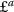-functions are the Fourier images of all square summable functions supported on $$[-a,a]$$.

The following lemma can be derived from  and .

### Lemma 2.2

If $$q_{j}\in L^{2}(0,1/2)$$, then the sequences $$\{ \lambda _{n}\}_{-\infty,n\neq0}^{\infty}$$, $$\{\mu_{j,n}^{\pm}\}_{-\infty ,n\neq 0}^{\infty}\cup\{\mu_{j,+0}^{\pm}\}\cup\{\mu_{j,-0}^{\pm}\}$$ and $$\{\nu_{j,n}^{\pm}\}_{-\infty,n\neq0}^{\infty}$$, which are the sets of zeros of the functions

\begin{aligned}& \omega(\lambda) =\cos\lambda+A\frac{\sin\lambda}{\lambda}+\frac{ g(\lambda)}{\lambda}, \end{aligned}
(2.19)
\begin{aligned}& \omega_{j}^{-}(\lambda,h_{\pm}) =-\lambda\sin \frac{\lambda}{2}+(h_{\pm}+A_{j})\cos \frac{\lambda}{2}+f_{j}^{\pm}(\lambda), \end{aligned}
(2.20)
\begin{aligned}& \omega_{j}^{+}(\lambda,h_{\pm}) =\cos \frac{\lambda }{2}+(A_{j}-h_{\pm}) \frac{\sin\frac{\lambda}{2}}{\lambda}+ \frac{g_{j}^{\pm}(\lambda)}{\lambda}, \end{aligned}
(2.21)

behave asymptotically as follows:

\begin{aligned}& \lambda_{n} = \biggl( n-\frac{1}{2} \biggr) \pi+ \frac{A}{(n-1/2)\pi }+\frac{\alpha_{n}}{n}, \end{aligned}
(2.22)
\begin{aligned}& \mu_{j,n}^{\pm} =2n\pi+\frac{h_{\pm}+A_{j}}{n\pi}+\frac{\alpha _{n}}{n}, \end{aligned}
(2.23)
\begin{aligned}& \nu_{j,n}^{\pm} =(2n-1)\pi+\frac{A_{j}-h_{\pm}}{(n-1/2)\pi}+ \frac{ \alpha_{n}}{n}. \end{aligned}
(2.24)

Here $$A=A_{1}+A_{2}$$, $$A_{j}=\frac{1}{2}\int_{0}^{1/2}q_{j}(t)\mathrm{d}\,t$$, $$h_{\pm}\in\mathbb{R}$$, the functions $$g(\lambda)$$ and $$g_{j}^{\pm }(\lambda)$$ are odd, $$f_{j}^{\pm}(\lambda)$$ are even,,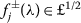and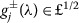, the sequences $$\{\alpha_{n}\}$$ are different in (2.22)(2.24) but all belong to $$l^{2}$$.

### Lemma 2.3

If $$h_{-}>h_{+}$$, then the eigenvalues $$\{\mu_{j,n}^{\pm }\}_{-\infty,n\neq0}^{\infty}\cup\{\mu_{j,+0}^{\pm}\}\cup\{\mu _{j,-0}^{\pm}\}$$ and $$\{\nu_{j,n}^{\pm}\}_{-\infty,n\neq0}^{\infty}$$ satisfy the following interlacing property:

\begin{aligned}& -\infty< \bigl(\mu_{j,+0}^{+}\bigr)^{2}< \bigl( \mu_{j,+0}^{-}\bigr)^{2}< \bigl(\mu _{j,1}^{+}\bigr)^{2}< \bigl(\mu_{j,1}^{-} \bigr)^{2}< \cdots; \\& -\infty< \bigl(\nu_{j,1}^{-}\bigr)^{2}< \bigl( \nu_{j,1}^{+}\bigr)^{2}< \bigl(\nu _{j,2}^{-}\bigr)^{2}< \bigl(\nu _{j,2}^{+} \bigr)^{2}< \cdots. \end{aligned}

### Proof

The proof refers to [2, Theorem 4.4.3] and is therefore omitted. □

### Definition 2.4

([6, Definition 2.3] or )

A meromorphic function $$f(z)$$ is said to be an essentially positive Nevanlinna function if

1. (1)

$$\operatorname{Im} z \operatorname{Im} f(z)>0$$ for all nonreal z,

2. (2)

there exists $$\beta\in\mathbb{R}$$ such that $$f(z)>0$$ for all $$z<\beta$$.

### Lemma 2.5

Suppose $$h_{-}>h_{+}$$. The eigenvalues $$\{\lambda _{n}\}_{-\infty,n\neq0}^{\infty}$$ of the problem (2.4)(2.8) are interlaced with the union $$\{\theta_{n}\}_{-\infty,n\neq 0}^{\infty }\cup\{\theta_{+0}\}\cup\{\theta_{-0}\}:=\{\mu_{1,n}^{-}\} _{-\infty ,n\neq0}^{\infty}\cup\{\mu_{1,+0}^{-}\}\cup\{\mu_{1,-0}^{-}\}\cup \{\nu_{2,n}^{-}\}_{-\infty,n\neq0}^{\infty}$$ in the following sense:

$$-\infty< \theta_{+0}^{2}\leq\lambda_{1}^{2} \leq\theta_{1}^{2}\leq \lambda_{2}^{2} \leq\cdots.$$
(2.25)

Moreover, $$\lambda_{n}=\theta_{n-1}$$ if and only if $$\lambda _{n}=\theta _{n}$$ for all $$n>0$$.

### Proof

We firstly show that $$\omega_{1}^{-}(\sqrt{z},h_{-})/\omega _{1}^{-}(\sqrt{z},h_{+})$$ and $$\omega_{2}^{+}(\sqrt{z},h_{+})/\omega_{2}^{+}(\sqrt{z},h_{-})$$ are essentially positive Nevanlinna functions. Since the zeros of the entire functions $$\omega_{1}^{-}(\sqrt{z},h_{-})$$ and $$\omega _{1}^{-}(\sqrt{z},h_{+})$$ are $$\{(\mu_{1,n}^{-})^{2}\}_{n=0}^{\infty}$$ and $$\{ (\mu _{1,n}^{+})^{2}\}_{n=0}^{\infty}$$, respectively, it follows from (2.20) and (2.23) that

$$\omega_{1}^{-}(\sqrt{z},h_{-})=C_{1} \prod_{n=0}^{\infty} \biggl( 1-\frac{z}{(\mu_{1,n}^{-})^{2}} \biggr) \quad \text{and} \quad \omega _{1}^{-}( \sqrt{z},h_{+})=C_{2}\prod_{n=0}^{\infty} \biggl( 1-\frac{z}{(\mu_{1,n}^{+})^{2}} \biggr) ,$$

where

$$C_{1}=\frac{(\mu_{1,+0}^{-})^{2}}{2}\prod_{n=1}^{\infty} \frac{(\mu _{1,n}^{-})^{2}}{(2n\pi)^{2}}\quad \text{and } \quad C_{2}=\frac{(\mu_{1,+0}^{+})^{2}}{2}\prod _{n=1}^{\infty}\frac{(\mu _{1,n}^{+})^{2}}{(2n\pi)^{2}}.$$

The condition $$h_{-}>h_{+}$$ and Lemma 2.3 imply that

$$-\infty< \bigl(\mu_{1,+0}^{+}\bigr)^{2}< \bigl( \mu_{1,+0}^{-}\bigr)^{2}< \bigl(\mu _{1,1}^{+}\bigr)^{2}< \bigl(\mu_{1,1}^{-} \bigr)^{2}< \cdots.$$

This together with [4, Theorem 2.1] or [20, Theorem 1, p.308] shows that the function

$$f(z):=\frac{\omega_{1}^{-}(\sqrt{z},h_{-})}{\omega_{1}^{-}(\sqrt {z},h_{+})}=C\prod_{n=0}^{\infty} \biggl( 1-\frac{z}{(\mu_{1,n}^{-})^{2}} \biggr) \biggl( 1-\frac{z}{(\mu_{1,n}^{+})^{2}} \biggr) ^{-1}$$

is a meromorphic function with Imz Im$$f(z)>0$$ for all nonreal z, since the constant $$C=C_{1}/C_{2}>0$$. We have from (2.20)

$$\lim_{z\rightarrow-\infty}\omega_{1}^{-}( \sqrt{z},h_{\pm})=\frac {\sqrt{|z|}}{2}\bigl(e^{\frac{\sqrt{|z|}}{2}}-e^{-\frac{\sqrt{|z|}}{2}} \bigr) \bigl(1+o(1)\bigr),$$
(2.26)

which means there exists $$\beta\in\mathbb{R}$$ such that $$f(z)>0$$ for all $$z<\beta$$. Thus the function $$\omega_{1}^{-}(\sqrt{z},h_{-})/\omega _{1}^{-}(\sqrt{z},h_{+})$$ is an essentially positive Nevanlinna function. For the same reason, one can easily verify that $$\omega_{2}^{+}(\sqrt {z},h_{+})/\omega_{2}^{+}(\sqrt{z},h_{-})$$ is also an essentially positive Nevanlinna function.

Moreover, we find from [19, Remark 5.1.21] that if f is a meromorphic function which satisfies condition (1) of Definition 2.4, then such is also $$-f^{-1}$$. Therefore the function $$-\omega _{1}^{-}(\sqrt{z},h_{+})/\omega_{1}^{-}(\sqrt{z},h_{-})$$ is also a meromorphic function that satisfies condition (1) of Definition 2.4. It follows from (2.18) that

$$\frac{(h_{-}-h_{+})\omega(\sqrt{z})}{\omega_{1}^{-}(\sqrt {z},h_{-})\omega _{2}^{+}(\sqrt{z},h_{-})}=\frac{\omega_{2}^{+}(\sqrt{z},h_{+})}{\omega _{2}^{+}(\sqrt{z},h_{-})}-\frac{\omega_{1}^{-}(\sqrt{z},h_{+})}{\omega _{1}^{-}(\sqrt{z},h_{-})},$$
(2.27)

which means the meromorphic function $$\frac{(h_{-}-h_{+})\omega(\sqrt {z})}{\omega_{1}^{-}(\sqrt{z},h_{-})\omega_{2}^{+}(\sqrt{z},h_{-})}$$ satisfies condition (1) of Definition 2.4 after cancellation of common factors in the numerator and in the denominator. Moreover, it follows from (2.19) and (2.21) that

$$\lim_{z\rightarrow-\infty}\omega(\sqrt{z})=\frac{e^{\sqrt {|z|}}+e^{-\sqrt{|z|}}}{2}\bigl(1+o(1) \bigr)$$

and

$$\lim_{z\rightarrow-\infty}\omega_{2}^{+}( \sqrt{z},h_{\pm})=\frac {1}{2}\bigl(e^{\frac{\sqrt{|z|}}{2}}+e^{-\frac{\sqrt{|z|}}{2}} \bigr) \bigl(1+o(1)\bigr),$$

which together with $$h_{-}>h_{+}$$ and (2.26) yield $$\frac{(h_{-}-h_{+})\omega(\sqrt{z})}{\omega_{1}^{-}(\sqrt{z},h_{-})\omega _{2}^{+}(\sqrt{z},h_{-})}>0$$ for $$z\rightarrow-\infty$$. Thus we have showed that the function $$\frac{(h_{-}-h_{+})\omega(\sqrt{z})}{\omega _{1}^{-}(\sqrt{z},h_{-})\omega_{2}^{+}(\sqrt{z},h_{-})}$$ is an essentially positive Nevanlinna function, and hence we get from [19, Remark 5.2.1 and Corollary 5.2.3] that

$$-\infty< \theta_{+0}^{2}\leq\lambda_{1}^{2} \leq\theta_{1}^{2}\leq \lambda_{2}^{2}< \cdots.$$

Suppose $$\lambda_{n}=\theta_{n}$$, then $$\omega(\lambda_{n})=0$$ and either $$\omega_{1}^{-}(\theta_{n},h_{-})=0$$ or $$\omega_{2}^{+}(\theta _{n},h_{-})=0$$. If $$\omega_{1}^{-}(\theta_{n},h_{-})=0$$, then it follows from Lemma 2.3 and (2.18) that $$\omega_{1}^{-}(\theta _{n},h_{+})\neq0$$ and $$\omega_{1}^{-}(\theta_{n},h_{+})\omega _{2}^{+}(\theta_{n}, h_{-})=0$$. This shows that $$\omega_{2}^{+}(\theta _{n},h_{-})=0$$, i.e. $$\theta_{n}$$ is a multiple eigenvalue of $$\omega _{1}^{-}(\lambda,h_{-})\omega_{2}^{+}(\lambda,h_{-})$$, which implies that $$\theta_{n}=\theta_{n-1}$$. On the other hand, since the eigenvalues $$\lambda_{n}$$ are simple, we find from (2.25) that the maximal multiplicity of $$\theta_{n}$$ is two. The proof is complete. □

### Lemma 2.6

Suppose $$h_{-}>h_{+}$$. The eigenvalues $$\{\lambda _{n}\}_{-\infty,n\neq0}^{\infty}$$ of the problem (2.4)(2.8) are interlaced with the union $$\{\zeta_{n}\}_{-\infty,n\neq0}^{\infty }\cup\{\zeta_{+0}\}\cup\{\zeta_{-0}\}:=$$ $$\{\mu_{1,n}^{-}\} _{-\infty ,n\neq0}^{\infty}\cup\{\mu_{1,+0}^{-}\}\cup\{\mu_{1,-0}^{-}\}\cup \{\nu_{2,n}^{+}\}_{-\infty,n\neq0}^{\infty}$$ as follows: for $$n\geq1$$ each interval $$(-\infty,\lambda_{n}^{2})$$ contains n or $$n-1$$ elements of the sequence $$\{\zeta_{n}^{2}\}_{n=0}^{\infty}$$.

### Proof

It follows from Lemma 2.5 that

$$\theta_{n-1}^{2}\leq\lambda_{n}^{2}\leq \theta_{n}^{2} \quad \mbox{for } n\geq1,$$

where $$\{\theta_{n}\}_{-\infty,n\neq0}^{\infty}\cup\{\theta_{+0}\} \cup \{\theta_{-0}\}=$$ $$\{\mu_{1,n}^{-}\}_{-\infty,n\neq0}^{\infty}\cup \{\mu_{1,+0}^{-}\}\cup\{\mu_{1,-0}^{-}\}\cup\{\nu_{2,n}^{-}\} _{-\infty ,n\neq0}^{\infty}$$. Moreover, we find from Lemma 2.3 that for two sequences $$\{\theta_{n}^{2}\}_{n=0}^{\infty}$$ and $$\{\zeta _{n}^{2}\}_{n=0}^{\infty}$$:

$$\theta_{n-1}^{2}\leq\zeta_{n-1}^{2}\leq \theta_{n}^{2} \quad \mbox{for } n\geq1.$$

There are two cases to be considered. Case (I): $$\theta _{n-1}^{2}=\theta _{n}^{2}$$, then we must have

$$\lambda_{n-1}^{2}< \theta_{n-1}^{2}= \lambda_{n}^{2}=\theta _{n}^{2}\quad \text{and}\quad \zeta_{n-2}^{2}< \theta_{n-1}^{2}= \zeta _{n-1}^{2}=\theta_{n}^{2}\quad ( \text{if any}),$$

which means $$(-\infty,\lambda_{n}^{2})$$ contains $$n-1$$ elements of the sequence $$\{\zeta_{n}^{2}\}_{n=0}^{\infty}$$. Case (II): $$\theta _{n-1}^{2}<\theta_{n}^{2}$$. Therefore if $$\zeta_{n-1}^{2}\in[\theta _{n-1}^{2},\lambda_{n}^{2})$$, then $$(-\infty,\lambda_{n}^{2})$$ contains n elements of the sequence $$\{\zeta_{n}^{2}\}_{n=0}^{\infty}$$; if $$\zeta _{n-1}^{2}\in{}[\lambda_{n}^{2},\theta_{n}^{2}]$$, then $$(-\infty ,\lambda_{n}^{2})$$ contains $$n-1$$ elements of the sequence $$\{\zeta _{n}^{2}\}_{n=0}^{\infty}$$. The proof is complete. □

## 3 Inverse three spectra problem

In this section, we shall solve the inverse spectral problem, give the sufficient conditions for three sequences to be the spectra of $$L(0,1;0,\infty;q)$$, $$L(0,1/2;0,h_{-};q)$$ and $$L(1/2,1;h_{+},\infty;q)$$ with $$h_{-}\neq h_{+}$$, and further describe the procedure of recovering the potential.

The main result of this paper is given as follows.

### Theorem 3.1

Let $$\{\lambda_{n}\}_{-\infty,n\neq0}^{\infty}$$ ($$\lambda_{-n}=-\lambda_{n}$$), $$\{\mu_{1,n}^{-}\}_{-\infty,n\neq 0}^{\infty}\cup\{\mu_{1,+0}^{-}\}\cup\{\mu_{1,-0}^{-}\}$$ ($$\mu _{1,-n}^{-}=-\mu_{1,n}^{-}$$) and $$\{\nu_{2,n}^{+}\}_{-\infty,n\neq 0}^{\infty}$$ ($$\nu_{2,-n}^{+}=-\nu_{2,n}^{+}$$) be three sequences of real and pure imaginary numbers with $$\lambda_{k}^{2}<\lambda_{k^{\prime }}^{2}$$, $$(\mu_{1,k}^{-})^{2}<(\mu_{1,k^{\prime}}^{-})^{2}$$ and $$(\nu _{2,k}^{+})^{2}<(\nu_{2,k^{\prime}}^{+})^{2}$$ for $$k< k^{\prime}$$. Suppose the following conditions are valid:

1. (1)

$$\{\lambda_{n}\}_{-\infty,n\neq0}^{\infty}$$ satisfy (2.22), $$\{\mu_{1,n}^{-}\}_{-\infty,n\neq0}^{\infty}\cup\{\mu _{1,+0}^{-}\}\cup\{\mu_{1,-0}^{-}\}$$ satisfy (2.23) with $$j=1$$, and $$\{\nu_{2,n}^{+}\}_{-\infty,n\neq0}^{\infty}$$ satisfy (2.24) with $$j=2$$, where A, $$A_{j}$$ and $$h_{\pm}$$ are all real numbers with $$A=A_{1}+A_{2}$$ and $$h_{-}\neq h_{+}$$.

2. (2)
$$-\infty< \zeta_{+0}^{2}< \lambda_{1}^{2}< \zeta_{1}^{2}< \lambda _{2}^{2}< \cdots,$$

where $$\{\zeta_{n}\}_{-\infty,n\neq0}^{\infty}\cup\{\zeta_{+0}\} \cup \{\zeta_{-0}\}:= \{\mu_{1,n}^{-}\}_{-\infty,n\neq0}^{\infty}\cup \{\mu_{1,+0}^{-}\}\cup\{\mu_{1,-0}^{-}\}\cup\{\nu_{2,n}^{+}\} _{-\infty ,n\neq0}^{\infty}$$.

Then there exists a unique pair of real functions $$q_{j}(x)\in L_{2}(0,1/2)$$ ($$j=1,2$$) which generate problem (2.4)(2.8) with the spectrum $$\{\lambda_{n}\}_{-\infty,n\neq0}^{\infty}$$, problem (2.10) with $$j=1$$ and the spectrum $$\{\mu_{1,n}^{-}\}_{-\infty,n\neq 0}^{\infty}\cup\{\mu_{1,+0}^{-}\}\cup\{\mu_{1,-0}^{-}\}$$, problem (2.14) with $$j=2$$ and the spectrum $$\{\nu_{2,n}^{+}\}_{-\infty,n\neq 0}^{\infty}$$.

In order to prove our main result, we need the following lemma.

### Lemma 3.2

The set of zeros of the function

$$\Phi(\lambda)=-\lambda\sin\frac{\lambda}{2}\cos\frac{\lambda}{2}+(A_{1}+h_{+}) \cos^{2}\frac{\lambda}{2}-(A_{2}-h_{-}) \sin^{2}\frac{\lambda}{2}+f(\lambda),$$
(3.1)

can be given as the union of two sets (denoted by $$\{\xi_{1,n}\} _{-\infty ,n\neq0}^{\infty}\cup\{\xi_{1,+0}\}\cup\{\xi_{1,-0}\}$$ and $$\{\xi _{2,n}\}_{-\infty,n\neq0}^{\infty}$$) such that $$\xi_{j,-n}=-\xi_{j,n}$$ and

\begin{aligned}& \xi_{1,n} =2n\pi+\frac{A_{1}+h_{+}}{n\pi}+\frac{\alpha_{n}}{n}, \end{aligned}
(3.2)
\begin{aligned}& \xi_{2,n} =(2n-1)\pi+\frac{A_{2}-h_{-}}{(n-1/2)\pi}+\frac{\alpha _{n}}{n}, \end{aligned}
(3.3)

where $$A_{j}$$ and $$h_{\pm}$$ are real constants, f is an even function and, the sequences $$\{\alpha_{n}\}$$ are different in (3.2)(3.3) but all belong to $$l^{2}$$.

### Proof

It follows from (3.1) that the function $$\Phi(\lambda)$$ can be rewritten as

$$\Phi(\lambda)=\Phi_{0}(\lambda)+f_{0}(\lambda),$$
(3.4)

whereand

\begin{aligned} \Phi_{0}(\lambda) =& -\lambda\sin\frac{\lambda}{2}\cos \frac {\lambda}{2}+(A_{1}+h_{+})\cos^{2} \frac{\lambda}{2}-(A_{2}-h_{-})\sin^{2} \frac{\lambda}{2} \\ &{} +(A_{1}+h_{+}) (A_{2}-h_{-}) \frac{\sin\frac{\lambda}{2}\cos\frac {\lambda }{2}}{\lambda} \\ =& \biggl[ -\lambda\sin\frac{\lambda}{2}+(A_{1}+h_{+}) \cos\frac {\lambda}{2} \biggr] \biggl[ \cos\frac{\lambda}{2}+\frac{A_{2}-h_{-}}{\lambda } \sin \frac{\lambda}{2} \biggr] . \end{aligned}

Denote

$$C_{n}(\delta)= \biggl\{ \lambda: \biggl\vert \lambda- \biggl( 2n \pi+\frac{ A_{1}+h_{+}}{n\pi} \biggr) \biggr\vert =\frac{\delta}{n} \biggr\} \quad \text{with }\delta>0.$$

Then, for $$\lambda\in C_{n}(\delta)$$, we have

$$\lambda=2n\pi+\frac{A_{1}+h_{+}}{n\pi}+\frac{\delta}{n}e^{i\theta},\quad \theta \in{}[0,2\pi),$$

which implies that

\begin{aligned}& \sin \biggl( n\pi+\frac{A_{1}+h_{+}}{2n\pi}+\frac{\delta }{2n}e^{i\theta } \biggr) =(-1)^{n} \biggl( \frac{A_{1}+h_{+}}{2n\pi}+\frac{\delta}{2n} e^{i\theta} \biggr) +O \biggl( \frac{1}{n^{3}} \biggr) , \\& \cos \biggl( n\pi+\frac{A_{1}+h_{+}}{2n\pi}+\frac{\delta }{2n}e^{i\theta } \biggr) =(-1)^{n}+O \biggl( \frac{1}{n^{2}} \biggr) , \\& \Phi_{0} \biggl( 2n\pi+\frac{A_{1}+h_{+}}{n\pi}+\frac{\delta }{n}e^{i\theta } \biggr) =-\delta\pi e^{i\theta}+O \biggl( \frac{1}{n^{2}} \biggr) . \end{aligned}

Therefore, for any $$\epsilon\in(0,\delta)$$ and any $$\theta\in {}[ 0,2\pi)$$ there exists $$N(\epsilon)$$ such that for $$n>N(\epsilon)$$

$$\biggl\vert \Phi_{0} \biggl( 2n\pi+\frac{A_{1}+h_{+}}{n\pi}+ \frac {\delta}{n}e^{i\theta} \biggr) \biggr\vert >\delta\pi- \epsilon.$$

Moreover, we have from [1, Lemma 1.4.3]

$$\lim_{n\rightarrow\infty}f_{0} \biggl( 2n\pi+\frac{A_{1}+h_{+}}{n\pi}+ \frac{\delta}{n}e^{i\theta} \biggr) =0$$

uniformly with respect to $$\theta\in{}[0,2\pi)$$. Thus, we conclude that for sufficiently large n ($$n>N_{1}>N(\epsilon)$$)

\begin{aligned} & \biggl\vert f_{0} \biggl( 2n\pi+\frac{A_{1}+h_{+}}{n\pi}+ \frac{\delta }{n}e^{i\theta} \biggr) \biggr\vert \\ &\quad < \delta\pi-\epsilon< \biggl\vert \Phi_{0} \biggl( 2n \pi+\frac{A_{1}+h_{+}}{n\pi}+\frac{\delta}{n}e^{i\theta} \biggr) \biggr\vert . \end{aligned}

It is obvious that the set of zeros of $$\Phi_{0}(\lambda)$$ consists of two subsequences and one of these subsequences due to its asymptotics has exactly one element inside each circle $$C_{n}(\delta)$$ for $$n>N_{1}$$. Hence we conclude by Rouche’s theorem that, for sufficiently large n, there is exactly one zero of $$\Phi(\lambda)$$ inside circle $$C_{n}(\delta)$$. Since $$\delta>0$$ is arbitrary, there is a subsequence of zeros of $$\Phi (\lambda)$$ of the form

$$\xi_{1,n}=2n\pi+\frac{A_{1}+h_{+}}{n\pi}+\frac{\delta_{n}}{n},$$
(3.5)

where $$\delta_{n}=o(1)$$. Substituting (3.5) into (3.1), using the condition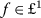and [18, Theorem 17 and Remark, pp. 82–83], we obtain $$\{\delta_{n}\}\in l^{2}$$, which means (3.2) is obtained. In the same way we can obtain the asymptotics for $$\{\xi _{2,n}\}_{-\infty,n\neq0}^{\infty}$$. The proof is complete. □

We are now in a position to give the proof of Theorem 3.1.

### Proof of Theorem 3.1

Without loss of generality, we always assume $$h_{-}> h_{+}$$. Using (2.22)–(2.24) we find

\begin{aligned}& A =\lim_{n\rightarrow\infty}(n-1/2)\pi \bigl( \lambda_{n}-(n-1/2) \pi \bigr) , \end{aligned}
(3.6)
\begin{aligned}& h_{-}+A_{1} =\lim_{n\rightarrow\infty}n\pi \bigl( \mu _{1,n}^{-}-2n\pi \bigr) , \end{aligned}
(3.7)
\begin{aligned}& A_{2}-h_{+} =\lim_{n\rightarrow\infty}(n-1/2)\pi \bigl( \nu _{2,n}^{+}-(2n-1)\pi \bigr) , \end{aligned}
(3.8)

which imply that $$h_{-}-h_{+}=(h_{-}+A_{1})+(A_{2}-h_{+})-A$$. Consider the functions

\begin{aligned}& P(\lambda) =\prod_{n=1}^{\infty} \frac{\lambda_{n}^{2}-\lambda^{2}}{(n-1/2)^{2}\pi^{2}}, \\& P_{1}(\lambda) =\frac{(\mu_{1,+0}^{-})^{2}-\lambda^{2}}{2}\prod _{n=1}^{\infty}\frac{(\mu_{1,n}^{-})^{2}-\lambda^{2}}{(2n\pi )^{2}}, \\& P_{2}(\lambda) =\prod_{n=1}^{\infty} \frac{(\nu _{2,n}^{+})^{2}-\lambda^{2}}{(2n-1)^{2}\pi^{2}}. \end{aligned}

Then we have from [1, Lemma 3.4.2]

\begin{aligned}& P(\lambda) =\cos\lambda+A\frac{\sin\lambda}{\lambda}+\frac{g_{0}(\lambda)}{\lambda}, \end{aligned}
(3.9)
\begin{aligned}& P_{1}(\lambda) =-\lambda\sin\frac{\lambda}{2}+(h_{-}+A_{1}) \cos \frac{\lambda}{2}+g_{1}(\lambda), \end{aligned}
(3.10)
\begin{aligned}& P_{2}(\lambda) =\cos\frac{\lambda}{2}+(A_{2}-h_{+}) \frac{\sin\frac{ \lambda}{2}}{\lambda}+\frac{g_{2}(\lambda)}{\lambda}, \end{aligned}
(3.11)

where,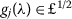for $$j=1,2$$. It follows directly from the infinite product representation of $$P(\lambda)$$ and $$P_{j}(\lambda)$$ ($$j=1,2$$) that $$P(\lambda)$$ and $$P_{j}(\lambda)$$ are all even functions, so we find from (3.9)–(3.11) that $$g_{0}(\lambda)$$ and $$g_{2}(\lambda)$$ are odd, whereas $$g_{1}(\lambda)$$ is even. This makes $$g_{0}(0)=0=g_{2}(0)$$, which means $$g_{0}(\lambda)/\lambda$$ and $$g_{2}(\lambda)/\lambda$$ are entire functions. Define

$$\Phi(\lambda):=P_{1}(\lambda)P_{2}(\lambda)-(h_{-}-h_{+})P( \lambda).$$
(3.12)

It follows from (3.9)–(3.11) that the function $$\Phi (\lambda)$$ given by (3.12) can be represented as in (3.1). Therefore, we find from Lemma 3.2 that the set of zeros of $$\Phi(\lambda)$$ consists of two subsequences which we denote by $$\{\mu_{1,n}^{+}\} _{-\infty ,n\neq0}^{\infty}\cup\{\mu_{1,+0}^{+}\}\cup\{\mu_{1,-0}^{+}\}$$ and $$\{\nu_{2,n}^{-}\}_{-\infty,n\neq0}^{\infty}$$, which behave asymptotically as follows:

\begin{aligned}& \mu_{1,n}^{+} =2n\pi+\frac{A_{1}+h_{+}}{n\pi}+\frac{\alpha_{n}}{n}, \end{aligned}
(3.13)
\begin{aligned}& \nu_{2,n}^{-} =(2n-1)\pi+\frac{A_{2}-h_{-}}{(n-1/2)\pi}+ \frac {\alpha_{n}}{n}, \end{aligned}
(3.14)

where the constants $$A_{1}+h_{+}=A-(A_{2}-h_{+})$$ and $$A_{2}-h_{-}=A-(A_{1}+h_{-})$$ can be derived from (3.6)–(3.8), the sequences $$\{\alpha_{n}\}$$ are different in (3.13)–(3.14) but all belong to $$l^{2}$$.

Notice here that $$P_{1}(\lambda)P_{2}(\lambda)>0$$ and $$P(\lambda)>0$$ for $$\lambda^{2}\rightarrow-\infty$$. Condition (2) and the fact $$\Phi (\lambda _{n})=P_{1}(\lambda_{n})P_{2}(\lambda_{n})$$ yields

$$\Phi(\lambda_{n}) (-1)^{n}>0.$$

Condition (2), $$h_{-}>h_{+}$$ and the fact $$\Phi(\zeta _{n})=-(h_{-}-h_{+})P(\zeta_{n})$$ implies that

$$\Phi(\zeta_{n}) (-1)^{n+1}>0.$$

By direct calculation, we have from (3.9)–(3.12)

$$\lim_{\lambda^{2}\rightarrow-\infty}\Phi(\lambda)=\frac{|\lambda |}{2}\bigl(e^{|\lambda|}-e^{-|\lambda|}\bigr) \bigl(1+o(1)\bigr)= \frac{|\lambda|}{2}e^{|\lambda |}\bigl(1+o(1)\bigr),$$

which means $$\Phi(\lambda)>0$$ for $$\lambda^{2}\rightarrow-\infty$$. Taking into account the asymptotics (3.13)–(3.14), we conclude that each interval $$(-\infty,\zeta_{+0}^{2}), (\lambda _{1}^{2},\zeta _{1}^{2}), (\lambda_{2}^{2},\zeta_{2}^{2}),\ldots$$ , contains exactly one zero of the function $$\Phi(\sqrt{z})$$. Now we denote the zero of $$\Phi(\sqrt{z})$$ lying in $$(-\infty,\zeta_{+0}^{2})$$ by $$(\mu_{1,+0}^{+})^{2}$$ if $$\zeta_{+0}^{2}=(\mu_{1,+0}^{-})^{2}$$ and by $$(\nu _{2,1}^{-})^{2}$$ if $$\zeta_{+0}^{2}=(\nu_{2,1}^{+})^{2}$$. We denote the zero of $$\Phi (\sqrt{z})$$ lying in $$(\lambda_{n}^{2},\zeta_{n}^{2})$$ by $$(\mu _{1,k}^{+})^{2}$$ if $$\zeta_{n}^{2}=(\mu_{1,k}^{-})^{2}$$ and by $$(\nu _{2,k}^{-})^{2}$$ if $$\zeta_{n}^{2}=(\nu_{2,k}^{+})^{2}$$. Therefore, $$\{(\mu _{1,n}^{+})^{2}\}_{n=0}^{\infty}$$ interlace with $$\{(\mu _{1,n}^{-})^{2}\}_{n=0}^{\infty}$$ as:

$$-\infty< \bigl(\mu_{1,+0}^{+}\bigr)^{2}< \bigl( \mu_{1,+0}^{-}\bigr)^{2}< \bigl(\mu _{1,1}^{+}\bigr)^{2}< \bigl(\mu_{1,1}^{-} \bigr)^{2}< \cdots.$$
(3.15)

As is well known (see e.g. [1, p. 283]), two sequences $$\{(\mu _{1,n}^{+})^{2}\}_{n=0}^{\infty}$$ and $$\{(\mu _{1,n}^{-})^{2}\}_{n=0}^{\infty}$$ satisfying (2.23) with $$j=1$$ and (3.15) uniquely determine a real-valued function $$q_{1}\in L_{2}(0,1/2)$$, which generates the Neumann–Robin problem (2.10) for $$j=1$$ with the characteristic function $$\omega_{1}^{-}(\lambda,h_{-})$$ and the spectrum $$\{\mu_{1,n}^{-}\}_{-\infty,n\neq0}^{\infty}\cup\{\mu _{1,+0}^{-}\}\cup\{\mu_{1,-0}^{-}\}$$, and the Neumann–Robin problem (2.12) for $$j=1$$ with the characteristic function $$\omega _{1}^{-}(\lambda ,h_{+})$$ and the spectrum $$\{\mu_{1,n}^{+}\}_{-\infty,n\neq0}^{\infty }\cup\{\mu_{1,+0}^{+}\}\cup\{\mu_{1,-0}^{+}\}$$.

Now we need to find the constants $$h_{-}$$ and $$h_{+}$$. The entire functions $$\omega_{1}^{-}(\lambda,h_{-})$$ and $$\omega_{1}^{-}(\lambda,h_{+})$$ can be expressed as

$$\omega_{1}^{-}(\lambda,h_{-})= \frac{(\mu_{1,+0}^{-})^{2}-\lambda ^{2}}{2}\prod_{n=1}^{\infty} \frac{(\mu_{1,n}^{-})^{2}-\lambda^{2}}{(2n\pi )^{2}}\equiv P_{1}(\lambda)$$
(3.16)

and

$$\omega_{1}^{-}(\lambda,h_{+})= \frac{(\mu_{1,+0}^{+})^{2}-\lambda ^{2}}{2}\prod_{n=1}^{\infty} \frac{(\mu_{1,n}^{+})^{2}-\lambda^{2}}{(2n\pi )^{2}}.$$

Moreover, it follows from (2.11) and (2.13) for $$j=1$$ that

$$c_{1}(1/2,\lambda)=\frac{\omega_{1}^{-}(\lambda,h_{-})-\omega _{1}^{-}(\lambda,h_{+})}{h_{-}-h_{+}},$$
(3.17)

which together with the asymptotic expression for $$c_{1}(1/2,\lambda)$$ (see e.g. [6, Eq. (2.11)]) shows that

$$A_{1}=\frac{4\pi}{h_{-}-h_{+}}\lim_{n\rightarrow+\infty} \bigl\{ n \bigl[ \omega_{1}^{-}\bigl((4n+1)\pi,h_{-}\bigr)- \omega_{1}^{-}\bigl((4n+1)\pi ,h_{+}\bigr) \bigr] \bigr\} .$$
(3.18)

Thus, the parameters $$h_{-}=(h_{-}+A_{1})-A_{1}$$ and $$h_{+}=h_{-}-(h_{-}-h_{+})$$, which can be derived from (3.6)–(3.8) and (3.18).

We can find $$q_{1}(x)$$ via the procedure  described below. Without loss of generality let us assume that $$(\mu_{1,+0}^{+})^{2}>0$$, otherwise we apply a shift. Using the constant $$h_{-}$$, the functions $$\omega _{1}^{-}(\lambda,h_{-})$$ and $$c_{1}(1/2,\lambda)$$ (see (3.16) and (3.17)), we obtain

$$c_{1}^{\prime}(1/2,\lambda)=\omega_{1}^{-}( \lambda ,h_{-})-h_{-}c_{1}(1/2,\lambda),$$

further we construct $$e(\lambda)$$ as (see e.g. [1, p. 283] or [21, p. 390])

$$e(\lambda)=\bigl(c_{1}^{\prime}(1/2,\lambda)-i\lambda c_{1}(1/2,\lambda)\bigr) \mathrm{e}^{\frac{i\lambda}{2}},$$

which is the analog of the so-called Jost-function of the corresponding prolonged Sturm–Liouville problem on the half-axis $$[0,\infty)$$:

\begin{aligned}& -y^{\prime\prime}+q(x)y =\lambda^{2}y, x\in{}[0,\infty ), \\& y^{\prime}(0) =0, \end{aligned}

with

$$q(x)= \textstyle\begin{cases} q_{1}(x) &\text{for }x\in{}[0,1/2], \\ 0 &\text{for }x\in(1/2,\infty). \end{cases}$$

Construct the S-function of this problem on the half-axis thus:

$$S(\lambda)=\frac{e(-\lambda)}{e(\lambda)}.$$

Solving the Marchenko equation

$$K_{1}(x,y)+F(x+y)+ \int_{x}^{\infty}K_{1}(x,t)F(t+y)\, \mathrm{d}t=0,$$

where

$$F(x)=\frac{1}{2\pi} \int_{-\infty}^{\infty}\bigl(1-S(\lambda)\bigr) \mathrm{e}^{i\lambda x}\, \mathrm{d}\lambda,$$

we find the kernel $$K(x,y)$$ and the potential

$$q_{1}(x)=2\frac{\mathrm{d}K_{1}(x,x)}{\mathrm{d}x},$$

which is a real-valued function and belongs to $$L_{2}(0,1/2)$$. This potential generates the Neumann–Robin problem (2.10) for $$j=1$$ with the characteristic function $$\omega_{1}^{-}(\lambda,h_{-})\equiv P_{1}(\lambda )$$ and the spectrum $$\{\mu_{1,n}^{-}\}_{-\infty,n\neq0}^{\infty}\cup \{\mu_{1,+0}^{-}\}\cup\{\mu_{1,-0}^{-}\}$$, the Neumann–Robin problem (2.12) for $$j=1$$ with the characteristic function $$\omega _{1}^{-}(\lambda,h_{+})$$ and the spectrum $$\{\mu_{1,n}^{+}\}_{-\infty,n\neq0}^{\infty }\cup\{\mu_{1,+0}^{+}\}\cup\{\mu_{1,-0}^{+}\}$$.

In the same way we construct $$q_{2}(x)$$ using the sequences $$\{\nu _{2,n}^{+}\}_{-\infty,n\neq0}^{\infty}$$ and $$\{\nu_{2,n}^{-}\} _{-\infty ,n\neq0}^{\infty}$$. It is clear that the obtained $$q_{2}(x)$$ generated the Dirichlet–Robin problem (2.16) for $$j=2$$ with the characteristic function

$$\omega_{2}^{+}(\lambda,h_{-})=\prod _{n=1}^{\infty}\frac{(\nu _{2,n}^{-})^{2}-\lambda^{2}}{(2n-1)^{2}\pi^{2}},$$

and the Dirichlet–Robin problem (2.14) for $$j=2$$ with the characteristic function $$\omega_{2}^{+}(\lambda, h_{+})\equiv P_{2}(\lambda)$$.

It remains to prove that the spectrum of problem (2.4)–(2.8) generated by the obtained $$q_{1}$$ and $$q_{2}$$ coincides with $$\{\lambda _{n}\}_{-\infty,n\neq0}^{\infty}$$. Since the functions $$\omega _{1}^{-}(\lambda,h_{+})\omega_{2}^{+}(\lambda,h_{-})$$ and $$\Phi (\lambda)$$ have common zeros, there exists a constant C such that $$\Phi (\lambda)=C\omega_{1}^{-}(\lambda,h_{+})\omega_{2}^{+}(\lambda,h_{-})$$. We have from (2.20)–(2.21) and (3.1)

$$C=\lim_{\lambda^{2}\rightarrow-\infty}\frac{\Phi(\lambda)}{\omega _{1}^{-}(\lambda,h_{+})\omega_{2}^{+}(\lambda,h_{-})}=1,$$

that is, $$\Phi(\lambda)=\omega_{1}^{-}(\lambda,h_{+})\omega _{2}^{+}(\lambda,h_{-})$$. Hence we find from (2.18) and (3.12) that

\begin{aligned} \omega(\lambda)& =:\frac{1}{h_{-}-h_{+}}\bigl[\omega_{1}^{-}( \lambda ,h_{-})\omega_{2}^{+}(\lambda,h_{+})- \omega_{1}^{-}(\lambda ,h_{+})\omega _{2}^{+}(\lambda,h_{-})\bigr] \\ & =\frac{1}{h_{-}-h_{+}}\bigl[P_{1}(\lambda)P_{2}(\lambda)- \Phi(\lambda )\bigr] \\ & =P(\lambda) \end{aligned}

with the set of zeros $$\{\lambda_{n}\}_{-\infty,n\neq0}^{\infty}$$. The proof is complete. □

## 4 Conclusion

Inverse spectral problems consist in recovering operators from their spectral characteristics. Such problems play an important role in mathematics and have many applications in natural sciences. Our research focuses mainly on the inverse three spectra problems for the Sturm–Liouville operators, which have been studied fairly completely under the assumption that $$h_{-}= h_{+}$$. In this paper, we give the sufficient conditions for three sequences to be the spectra of problems (2.1), (2.2) and (2.3) with $$h_{-}\neq h_{+}$$, for which the uniqueness was studied in . We also present the algorithm of constructing the potential. Our result also holds for the special case of $$h_{-}=\infty$$ and $$h_{+}=0$$, thus it improves the result of Boyko et al. .

## References

1. Marchenko, V.A.: Sturm–Liouville Operators and Applications. Operator Theory: Advances and Applications, vol. 22. Birkhäuser, Basel (1986)

2. Zettl, A.: Sturm–Liouville Theory. Surveys and Monographs, vol. 121. Am. Math. Soc., Providence (2005)

3. Pivovarchik, V.: An inverse Sturm–Liouville problem by three spectra. Integral Equ. Oper. Theory 34, 234–243 (1999)

4. Gesztesy, F., Simon, B.: On the determination of a potential from three spectra. Am. Math. Soc. Transl. Ser. 2 189, 85–92 (1999)

5. Albeverio, S., Hryniv, R.O., Mykytyuk, Y.V.: Inverse spectral problems for coupled oscillating systems: reconstruction from three spectra. Methods Funct. Anal. Topol. 13, 110–123 (2007)

6. Boyko, O., Pivovarchik, V., Yang, C.-F.: On solvability of three spectra problem. Math. Nachr. 289, 1727–1738 (2016)

7. Boyko, O., Pivovarchik, V.: The inverse three-spectral problem for a Stieltjes string and the inverse problem with one-dimensional damping. Inverse Probl. 24, 015019 (2008)

8. Drignei, M.-C.: Constructibility of an $$L_{\mathbb{R}}^{2}(0,a)$$ solution to an inverse Sturm–Liouville problem using three Dirichlet spectra. Inverse Probl. 26, 025003 (2010)

9. Drignei, M.-C.: Numerical reconstruction in a three-spectra inverse Sturm–Liouville problem with mixed boundary conditions. Inverse Probl. Sci. Eng. 21, 1368–1391 (2013)

10. Drignei, M.-C.: Uniqueness of solutions to inverse Sturm–Liouville problems with $$L^{2}(0,a)$$ potential using three spectra. Adv. Appl. Math. 42, 471–482 (2009)

11. Fu, S., Xu, Z., Wei, G.: Inverse indefinite Sturm–Liouville problems with three spectra. J. Math. Anal. Appl. 381, 506–512 (2011)

12. Hryniv, R.O., Mykytyuk, Y.V.: Inverse spectral problems for Sturm–Liouville operators with singular potentials. Part III: reconstruction by three spectra. J. Math. Anal. Appl. 284, 626–646 (2003)

13. Michor, J., Teschl, G.: Reconstructing Jacobi matrices from three spectra: spectral methods for operators of mathematical physics. In: Spectral Methods for Operators of Mathematical Physics. Operator Theory: Advances and Applications, vol. 154, pp. 151–154. Birkhäuser, Basel (2004)

14. Pivovarchik, V.: A special case of the Sturm–Liouville inverse problem by three spectra: uniqueness results. Proc. R. Soc. Edinb., Sect. A 136, 181–187 (2006)

15. Pivovarchik, V., Woracek, H.: Sums of Nevanlinna functions and differential equations on star-shaped graphs. Oper. Matrices 3, 451–501 (2009)

16. Wei, G., Wei, X.: A generalization of three spectra theorem for inverse Sturm–Liouville problems. Appl. Math. Lett. 35, 41–45 (2014)

17. Boyko, O., Martinyuk, O., Pivovarchik, V.: Higher order Nevanlinna functions and the inverse three spectra problem. Opusc. Math. 36, 301–314 (2016)

18. Young, R.M.: An Introduction to Nonharmonic Fourier Series, revised first edn. Academic Press, New York (2001)

19. Möller, M., Pivovarchik, V.: Spectral Theory of Operator Pencils, Hermite–Biehler Functions, and Their Applications. Operator Theory: Advances and Applications, vol. 246. Birkhäuser, Basel (2015)

20. Levin, B.J.: Distribution of Zeros of Entire Functions, revised edn. Translations of Mathematical Monographs, vol. 5. Am. Math. Soc., Providence (1980)

21. Pivovarchik, V.: On the Hald–Gesztesy–Simon theorem. Integral Equ. Oper. Theory 73, 383–393 (2012)

### Acknowledgements

The authors would like to thank Professor V. Pivovarchik (South Ukrainian National Pedagogical University) for helpful suggestions and discussions, and would also like to thank the referees for their valuable comments, which improve and strengthen the presentation of this manuscript.

Not applicable.

## Funding

The research was supported in part by the NNSF (11601299, 11571212, 11471004), the China Postdoctoral Science Foundation (2016M600760) and the Fundamental Research Funds for the Central Universities (GK201701001).

## Author information

Authors

### Contributions

The authors have equal contributions to each part of this article. All the authors read and approved the final manuscript.

### Corresponding author

Correspondence to Guangsheng Wei.

## Ethics declarations

### Competing interests

The authors declare that they have no competing interests.

Not applicable.

### Publisher’s Note

Springer Nature remains neutral with regard to jurisdictional claims in published maps and institutional affiliations.

## Rights and permissions